# Henry's law

Henry's law is one of the gas laws, formulated by the British chemist, William Henry, in 1803. It states that:

At a constant temperature, the amount of a given gas dissolved in a given type and volume of liquid is directly proportional to the partial pressure of that gas in equilibrium with that liquid.

## Formula and Henry constant

A formula for Henry's law is: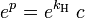$e^p = e^{k_{\rm H}}\;c$

where:$e\,$ is approximately 2.7182818, the base of the natural logarithm (also called Euler's number)$p\,$ is the partial pressure of the solute above the solution$c\,$ is the concentration of the solute in the solution (in one of its many units)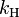$k_{\rm H}\,$ is the Henry's law constant, which has units such as L·atm/mol, atm/mole fraction or Pa·m3/mol.

Taking the natural logarithm of the formula, gives us the more commonly used formula:$p = k_{\rm H}\;c$

Some values for$k_{\rm H}\,$ include:

oxygen (O2) : 769.2 L·atm/mol
carbon dioxide (CO2) : 29.4 L·atm/mol
hydrogen (H2) : 1282.1 L·atm/mol

when these gases are dissolved in water at 298 kelvins.

As shown in Table 1 below, there are other forms of Henry's law each of which defines the constant$k_{\rm H}\,$ differently and requires different dimensional units. The form of the equation presented above is consistent with the example numerical values presented for oxygen, carbon dioxide and hydrogen and with their corresponding dimensional units.

Note that for the above values, the unit of concentration, c, was chosen to be molarity (i.e., mol/L). Hence the dimensional units: L is liters of solution, atm is the partial pressure of the gaseous solute above the solution (in atmospheres of absolute pressure), and mol is the moles of the gaseous solute in the solution. Also note that the Henry's law constant, kH, varies with the solvent and the temperature.

### Forms of Henry's law

There are various forms Henry's law which are discussed in the technical literature.

Table 1: Some forms of Henry's law and constants (gases in water at 298 K)
equation:$k_{\rm{H,pc}} = \frac{p_{\rm{gas}}}{c_{\rm {aq}}}$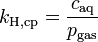$k_{\rm{H,cp}} = \frac{c_{\rm{aq}}}{p_{\rm{gas}}}$$k_{\rm{H,px}} = \frac{p_{\rm{gas}}}{x_{\rm{aq}}}$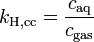$k_{\rm{H,cc}} = \frac{c_{\rm{aq}}}{c_{\rm{gas}}}$
dimension:$\left[\frac{\rm L_{\rm{soln}} \cdot \rm{atm}}{\rm{mol}_{gas}}\right]$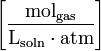$\left[\frac{\rm{mol}_{\rm{gas}}}{\rm{L}_{\rm{soln}} \cdot \rm{atm}}\right]$$\left[\frac{\rm{atm} \cdot \rm{mol}_{\rm{soln}}} {\rm{mol}_{\rm{gas}}}\right]$ dimensionless
O2 769.23 1.3 E-3 4.259 E4 3.180 E-2
H2 1282.05 7.8 E-4 7.099 E4 1.907 E-2
CO2 29.41 3.4 E-2 0.163 E4 0.8317
N2 1639.34 6.1 E-4 9.077 E4 1.492 E-2
He 2702.7 3.7 E-4 14.97 E4 9.051 E-3
Ne 2222.22 4.5 E-4 12.30 E4 1.101 E-2
Ar 714.28 1.4 E-3 3.955 E4 3.425 E-2
CO 1052.63 9.5 E-4 5.828 E4 2.324 E-2

where:$c_{\rm{aq}}\,$ = moles of gas per liter of solution$\mathrm{L}_{\rm{soln}}\,$ = liters of solution$p_{\rm{gas}}\,$ = partial pressure of gas above the solution, in atmospheres of absolute pressure$x_{\rm{aq}}\,$ = mole fraction of gas in solution = moles of gas per mole of solution ≈ moles of gas per mole of water$\rm{atm}\,$ = atmospheres of absolute pressure

As can be seen by comparing the equations in the above table, the Henry's law constant kH,pc is simply the inverse of the constant kH,cp. Since all kH may be referred to as the Henry's Law constant, readers of the technical literature must be quite careful to note which version of the Henry's law equation is being used.

It should also be noted the Henry's law is a limiting law that only applies for dilute solutions. The range of concentrations in which it applies becomes narrower the more the system diverges from ideal behavior. Roughly speaking, that is the more chemically different the solute is from the solvent.

It also only applies for solutions where the solvent does not react chemically with the gas being dissolved. A common example of a gas that does react with the solvent is carbon dioxide, which rapidly forms hydrated carbon dioxide and then carbonic acid (H2CO3) with water.

## Temperature dependence of Henry's law constant

When the temperature of a system changes, the Henry's law constant will also change. This is why some people prefer to name it Henry coefficient. There are multiple equations assessing the effect of temperature on the constant. This form of the van 't Hoff equation is one example: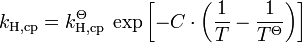$k_{\rm H,cp} = k_{\rm H,cp}^\Theta \; \exp \left[ -C \cdot \left( \frac{1}{T}-\frac{1}{T^\Theta}\right)\right]$
 where: T = any given temperature, in kelvins TΘ = the standard state temperature of 298 K kH,cp = the solubility form of Henry's law constant at the given temperature (in the units shown in Table 1)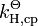$k_{\rm H,cp}^\Theta$ = the solubility form of Henry's law constant at TΘ exp = the exponential function C = a constant with dimension of kelvins

The above equation is an approximation only and should be used only when no better experimentally derived formula for a given gas exists.

The following table lists some values for constant C in the equation above:

 Gas O2 H2 CO2 N2 He Ne Ar CO C 1700 500 2400 1300 230 490 1300 1300

Because solubility of gases decreases with increasing temperature, the partial pressure a given gas concentration has in liquid must increase. While heating water (saturated with nitrogen) from 25 °C to 95 °C the solubility will decrease to about 43% of its initial value. Partial pressure of CO2 in seawater doubles with every 16 K increase in temperature.

The constant C may be regarded as:$C = \frac{\Delta_{\rm{\,solv}}\,H}{R} = \frac{-d \ln\left(k_{\rm{H,cp}} \right)}{d(1/T)}$
where: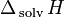$\Delta_{\rm{\,solv}}\,H$ is the enthalpy of solution R is the universal gas constant

## Henry's law in geophysics

In geophysics a version of Henry's law applies to the solubility of a noble gas in contact with silicate melt. One equation used is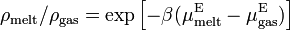$\rho_{\rm melt}/\rho_{\rm gas} = \exp\left[-\beta(\mu^{\rm E}_{\rm melt} - \mu^{\rm E}_{\rm gas})\right]\,$
 where: ρ = the densities of the solute gas in the melt and in the gas phases β =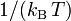$1/(k_{\rm B}\,T)\;$, an inverse temperature scale kB = the Boltzmann constant μE = the excess chemical potentials of the solute gas in the melt and in the gas phases

## Raoult's law compared to Henry's Law

In the mathematical expressions of the two laws, both state that the partial pressure of a component in a solution is proportional to the concentration of that component in the solution. Using mole fractions, x, as the expression of concentration, Henry's law can be written as: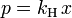$p = k_{\rm H}\,x$

This can be compared with Raoult's law: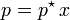$p = p^\star\,x$

where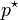$p^\star$ is the vapor pressure of the pure component.

Thus, Raoult's law appears to be a special case of Henry's law where kH is equal to$p^\star$. This is true for pairs of closely related substances, such as benzene and toluene, which obey Raoult's law over the entire composition range (such mixtures are called ideal mixtures).

The general case is that both laws are limit laws, and they apply at opposite ends of the composition range. The vapor pressure of the component with largest concentration by far, such as the solvent for a dilute solution, is proportional to the mole fraction, and the proportionality constant is the vapor pressure of the pure substance (Raoult's law).

The vapor pressure of component with the smallest concentration by far, such as the solute in a dilute solution, is also proportional to the mole fraction, but the proportionality constant is the Henry's law constant which must be determined experimentally (Henry's law).

In mathematical terms:

Raoult's law:$\lim_{x\to 1}\,(p/x) = p^\star$
Henry's law:$\lim_{x\to 0}\,(p/x) = k_{\rm H}$